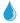課程地圖 CLASSINFO

(course name)
(中文)統計學特論
(Eng. )Special Topics on Statistics

(course no.)
以課程系統公告為主

(credits)
3

(dept. & year)
碩專一

(teacher)
陳鴻烈

(course type)
選修

(semester)
下學期

(course objectives)
(中文)做為學生今後學習其他科目之基礎工具。
(Eng. )Statistics is a basic tool to help students to learn other subjects in the future.

(course description)
(中文)使學生明瞭統計學原理應用在水土保持上的方法。
(Eng. )Let students understand how to use statistics in soil and water conservation research.

(course content and homework/tests schedule)

(week)

(subject and content)

(remark)
1
Introduction

2
Describing, Displaying, and Exploring Statistical Data

3
Statistical Process Control

4
Making Predictions: Regression Analysis

5
Statistical Analysis in Model Building

6
Probability

7
Probability (continue)

8
Random Variables and Probability Distributions

9
Important Probability Distributions in Engineering

10
Sampling Distributions

11
Sampling Distributions(continue)

12
Statistical Estimation

13
Statistical Estimation (continue)

14
Statistical Testing

15
Theory and Inferences in Regression Analysis

16
Analysis of Variance

17
Analysis of Variance (continue)

18
Experimental Design

(evaluation)
(中文)口頭報告、書面報告

(textbook)
(中文)Modern Engineering Statistics, Lawrence L. Lapin, Duxbury Press, 高立圖書有限公司.

(other references)
(中文)

v

v

v

v

v